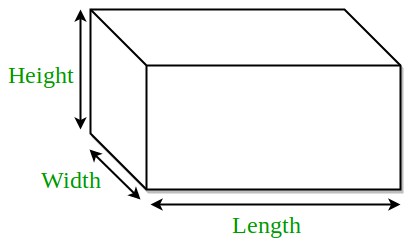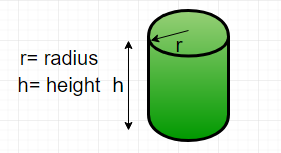# Surface Area of Cube, Cuboid and Cylinder | Class 8 Maths

• Last Updated : 03 Mar, 2021

In our daily life, we see many objects like books, pencil boxes, cones, football, cylinder. These all are three-dimensional objects (solid shapes). All these objects occupy some shape and have three dimensions Length, Breadth, Height, or Depth. We often find some shapes with two or more identical (congruent) faces. For example, the cube has squared faces on each side.

### Surface Area

The surface area of an object is the sum of all the areas of the shapes that cover the surface of the object. For example, the surface area of a cube is the sum of the area of six squares that cover it. If the area of one side is a2  then the area of six sides is 6a2 which is its surface area.

Hey! Looking for some great resources suitable for young ones? You've come to the right place. Check out our self-paced courses designed for students of grades I-XII

Start with topics like Python, HTML, ML, and learn to make some games and apps all with the help of our expertly designed content! So students worry no more, because GeeksforGeeks School is now here!

## Cube

Cube is a 3-dimensional box-like figure represented in the 3-dimensional plane. Cube has 6 squared-shape equal faces. Each face meets another face at 90 degrees each. Three sides of the cube meet at the same vertex.We know that the cube consists of 6 square faces. Let us consider, if each side of a cube is a, then the,

The total surface area of the cube is 6a2

### Length of Edge of Cube

From the surface area of the cube, we can find the length of the edge of the cube by rearranging the formula,

Area = 6 * side2

=> side2 = Area / 6

=> side = √Area / 6

### Examples

Example 1. If the sidewall of a cubic structure is 5m, then find the Total Surface Area?

Solution:

Given, length of sidewall = 5m

Total surface Area = 6a2

= 6 (52)

= 6 (25)

= 150 sq.m

Example 2. Find the length of the edge of the cube, if its Area is 294 sq.m?

Solution:

Given, Area = 294 sq.m

Length of edge of cube = √ Area / 6

= √ (294 / 6)

= √ 49

= 7 m

Example 3. What is the surface area of a cube of side 6cm?

Solution:

Given,

Side of cube = a = 6cm

Surface Area of cube = 6a2

= 6 * 62 cm2

= 216 cm2

Example 4. Find the side of a cube whose surface area is 384 cm2?

Solution:

Given,

Surface area of a cube = 384 cm2

Let ‘a’ be the side of a cube .

we know that , surface area of cube = 6a2

=> 6a2 = 384

=> a2  = 384 / 6

=> a2  =  64

=> a  = √64

=> a = 8

Therefore, side of a cube = 8cm.

Note: The lateral surface area of a cube is the Total surface area of a cube, which is equal to the sum of areas of all its sides.

## Cuboid

A cuboid is made up of six rectangular planes, with different values of length, width, and height. It may be a brick or a box which has a rectangular view from aside. A cuboid is a three-dimensional solid with six rectangular faces. It has 8 vertices and 12 edges. The opposite faces of cuboids are always equal.A cuboid has three measures Total surface area, Lateral surface area, and volume. The surface areas are measured in square units.

### Total Surface Area of a Cuboid

The Total Surface Area of a cuboid is the sum of its 6 rectangular faces, given by

2 (lw + wh + lh)

l = length, w = width, h = height

### Lateral Surface Area of a Cuboid

The Lateral Surface Area of a cuboid is the sum of its 4 rectangular faces leaving its base and top.

LSA = 2 (lh + wh)

= 2h (l + w) sq.units

### Examples

Example 1. Find the total surface area of a cuboid having dimensions as length = 4cm , width = 3cm , height = 2cm?

Solution:

Given h = 2cm, l = 4cm, w = 3cm

TSA = 2 (lw + wh + hl)

= 2 (4 * 3 + 3 * 2 + 2 * 4)

= 2 (12 + 6 + 8)

= 2 (26)

= 52 sq.cm

Example 2. Find the Lateral Surface area of the cuboid, if its length, breadth, and height are 8cm, 9cm, and 10cm respectively?

Solution:

Given length = 8cm

breadth = 9cm, height = 10cm

Lateral Surface Area = 2h (l + b)

= 2 * 10 (8 + 9)

= 2 * 10 (17)

= 2 * 170

= 340 cm2

Example 3. Find the Total and Lateral Surface Areas of the cuboid with the following dimensions?

Length = 4cm

width = 5cm

height = 6cm

Solution:

Total Surface Area = 2 (lw + wh + lh)

= 2 (4 * 5 + 5 * 6 + 4 * 6)

= 2 (20 + 30 + 24)

= 2 (74)

= 148 sq.cm

Lateral Surface Area = 2h (l + w)

= 2 * 6 (4 + 5)

= 12 (9)

= 108 sq.cm

## Cylinder

A cylinder is a three-dimensional object with two circular bases parallel to each other. Cylinder is a curved surface with two circular bases.• Curved Surface Area  of Cylinder = 2π * r * h sq.units,
• Area of two circular bases = 2 (πr2)

The Total Surface Area of a cylinder is the area occupied by its surface in three-dimensional space. It is defined as the sum of the area of curved surfaces and the Area of circular bases.

Total Surface Area of Cylinder = 2π * r * h + 2 (πr2)

= 2πr (h + r)

Where:

h = height of cylinder

π (pie) = 3.14 or 22/7

### Examples

Example 1. Find the curved surface area of the cylinder with a diameter of 6cm and a height of 4cm?

Solution:

Given,

diameter = 6cm , radius = 3cm, height = 4cm

Curved Surface Area = 2π * r * h Square Units.

= 2 * 3.14 * 3 * 4

= 75.36 cm2

Example 2. Calculate the cost required to paint a container that is in the shape of a cylinder having a base radius of 10cm and height of 15 cm. If the painting cost of the container is INR 3 / cm2?

Solution:

Given, Radius of container = 10cm

Height = 15cm

Total Surface Area of container = 2πr (h + r)

= 2 * 3.14 * 10 (15 + 10)

= 2 * 3.14 * 10 (25)

= 1570 cm2

Painting cost per 1cm2 = Rs. 3

Total cost of painting the container = 3 * 1570

= Rs.4710

Example 3. Find the total surface area and curved surface area of a cylinder with a diameter of 12cm and height of 10cm.

Solution:

Given,

diameter = 12cm, radius = 6cm

height = 10cm

Total Surface Area = 2πr (h + r)

= 2 * 3.14 * 6 (10 + 6)

= 602.88 sq.cm

Curved Surface Area =  2π * r * h

=  2π * 6 * 10

= 376.8 sq.cm

Example 4. If the lateral Surface Area of a cylinder is 484 cm2 and its height is 12 cm, then find its radius of the base?

Solution:

Given,

Lateral Surface Area of cylinder = 484

2π * r * h = 484

2 * 3.14 * r * 12 = 484

75.36 * r = 484

r = 484 / 75.36

r= 6.42

Therefore the radius of the cylinder is 6.42 cm.

My Personal Notes arrow_drop_up